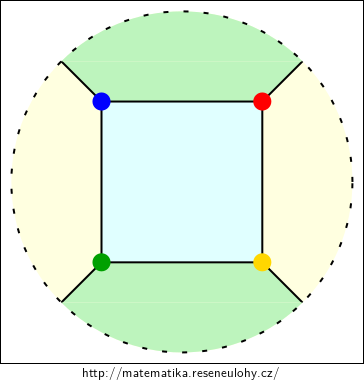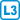Find a graph that can be drawn on a torus so that all faces are bounded by a four-cycle and have the chromatic number three.

• #### Hint

The graph can have an odd cycle as a subgraph, but not necessarily as a face.

• #### Solution

For example, the toroidal grid $$3 \times 3$$ contains a triangle, and it can be colored with three colors, see the picture.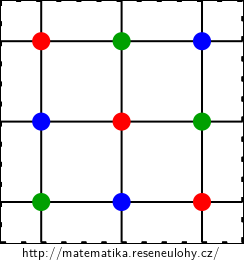• #### Variant

Try to find a graph with the same drawing conditions, but to have the chromatic number four.

• #### Hint

Try to start with the grid again, but this time wind it on the torus in a skewed way.

Algebraic tools, namely Cayley graphs , can be used to describe such a graph. Cayley graph for the group $$G$$ and the set of generators $$X \subseteq G$$ is a graph on $$| G |$$ vertices $$V$$, where each vertex corresponds to one element of the group $$G$$ and the edges correspond to the composition with generators.

In other words, there is a bijection $$f: V \rightarrow G$$ such that the vertices of $$u, v$$ are joined by an edge, iff there is $$x \in X$$ that $$f (u) x = f (v)$$ or $$f (u) x^{-1} = f (v)$$. The Cayley graph is usually denoted as $$Cay (G, X)$$.

Examples: $$C_5 = Cay (\mathbb Z_5, \{1 \})$$, $$K_5 = Cay (\mathbb Z_5, \{1{,}2 \})$$.

• #### Solution

Take the Cayley graph $$G = Cay (\mathbb Z_{13}, \{1{,}5 \})$$. This graph can be drawn on a torus with quadrangular faces.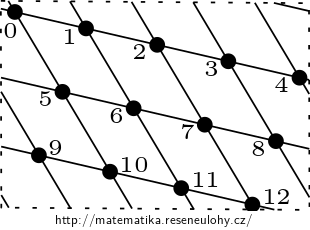It contains an odd cycle of 0-12, so its chromatic number is at least 3.

We show by a contradiction that the chromatic number is at least 4. If $$G$$ could be colored with three colors, one of the colors would have to be used at least five times. Without loss of generality, let it be red and let 0 be red. Red cannot be used on its neighbors, so four of the remaining eight vertices must be red. On these vertices we can find an eight-cycle 2-3-4-9-10-11-6-7, so either red are 2,4,10 and 6; or 3,9,11 and 7. The first option is excluded due to the edge $$(2{,}10)$$ the second due to $$(3{,}11)$$.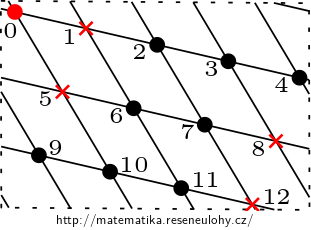However, coloring with four colors exists, indeed one of the colors needs to be used only once.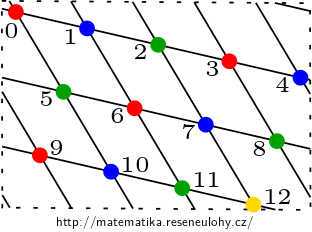• #### Variant

Can you find a graph that has a chromatic number four and whose drawing in the projective plane would have all the faces quadrangular?

• #### Solution

Just cleverly draw the graph $$K_4$$. It has three faces, they are marked in color in the picture.